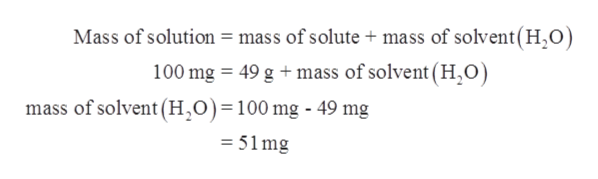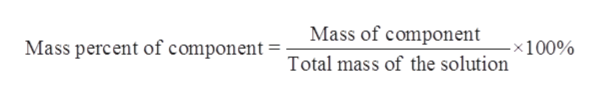# A 100 mg sample of Epsomite (MgSO4·7H2O) was heated in a crucible. After cooling to room temperature, the mass of the resulting white, anhydrous salt (MgSO4) was measured to be 49 mg. Given this data, calculate the mass percent of water in the hydrate and show calculations that confirm the empirical formula ratio of 1 MgSO4:7H2O.

Question
21 views

A 100 mg sample of Epsomite (MgSO4·7H2O) was heated in a crucible. After cooling to room temperature, the mass of the resulting white, anhydrous salt (MgSO4) was measured to be 49 mg. Given this data, calculate the mass percent of water in the hydrate and show calculations that confirm the empirical formula ratio of 1 MgSO4:7H2O.

check_circle

Step 1

It is given that:

Mass of Epsomite (MgSO4.7H2O) = 100 mg

Mass of anhydrous salt (MgSO4) = 49 mg

Step 2

Solution comprises of solute and solvent.

In this case mass of solution is 100 mg and mass of solute that is anhydrous salt (MgSO4) is 49 g. So, the mass of solvent can be calculated as follows:help_outlineImage TranscriptioncloseMass of solution = mass of solute + mass of solvent(H2O) 100 mg 49 gmass of solvent (H,0) mass of solvent (H,0)=100 mg - 49 mg =51mg fullscreen
Step 3

The way of representation of concentration of an element in a compound or a compone...help_outlineImage TranscriptioncloseMass of component Mass percent of component = x100% Total mass of the solution fullscreen

### Want to see the full answer?

See Solution

#### Want to see this answer and more?

Solutions are written by subject experts who are available 24/7. Questions are typically answered within 1 hour.*

See Solution
*Response times may vary by subject and question.
Tagged in

### Chemistry